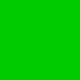# background subtraction of pointcloud

Is there any package for background subtraction of pointclouds?

I mean that "background subtraction" is the filter of pointcloud which doesn't change spatially compared with previous scene.

I want to use velodyne lider sensor, so I can't use depth image.

I know a PCL sample of this filter (http://pointclouds.org/documentation/tutorials/octree_change.php#octree-change-detection), and I can implement such package. However, if there is a filtering package, my implementation will come to nothing.

So I asked that anyone implemented such a package.

edit retag close merge delete

Please update your question rather than posting new answers on the same. It is more of good practice here for people who go through the answer later.

Sort by » oldest newest most votedhi, if you mean background subtraction as filtering then yes. PCL has filtering functions that you can use. But if you mean to subtract the table when u have objects of attention then there is no ready made package as such but you may go through the segmentation tutorial to get the cloud on top of the table and then process them. If you can specify what is the background in your case then we may be able to give a precise answer here.

Hope it helps!!!

Karthik

moreNot sure there is a package. I implemented a version of what you describe myself though using the 'RangeImagePlanar' point cloud type, as that preserves the locality information (x,y) of points and you only have to iterate over the image once this way. Don't have the code in a public repo, but here are the relevant bits (cobbled together, to give an idea on how one could do it quickly):

We fill a range image for every depth image coming from a RGB-D camera (given as depth_image_msg):

    range_image_planar->setDepthImage(reinterpret_cast<const float*> (&depth_image_msg->data),
depth_image_msg->width, depth_image_msg->height,
camera_info_msg->P,  camera_info_msg->P,
camera_info_msg->P,  camera_info_msg->P);



If we're in calibration mode, we update our reference image as needed (if the new distance value is closer or was invalid before we store that in the reference image):

  void calibrateReferenceImage(RangeImagePlanar* range_image_planar_in)
{
//if first image..
if(range_image_planar_reference == 0){
range_image_planar_reference = new RangeImagePlanar();
*range_image_planar_reference = *range_image_planar_in;
}else{
if ( pointcloudsSameSize(*range_image_planar_reference, *range_image_planar_in)){

int width_ref = range_image_planar_reference->width;
int height_ref = range_image_planar_reference->height;

for (int x = 0; x < width_ref; ++x){
for (int y = 0; y < height_ref; ++y){

if ( range_image_planar_in->isValid(x,y)){
const pcl::PointWithRange& tmpCurr ( range_image_planar_in->getPoint(x,y));

pcl::PointWithRange& tmpRef (range_image_planar_reference->getPoint(x,y) );

if ( (tmpRef.range > tmpCurr.range) || (tmpRef.range < 0.3)){
tmpRef = tmpCurr;
}
}
}
}
}else{
ROS_INFO("Reference and current cloud not same size! Not merging.\n");
}
}
}


For getting pointclouds of only the foreground we just compare the distance values between the current and the reference image:

int width_ref = range_image_planar_reference->width;
int height_ref = range_image_planar_reference->height;

pc_foreground_->clear();

//get Pointcloud with objects in foreground (into pc_foreground_)
for (int x = 0; x < width_ref; ++x){
for (int y = 0; y < height_ref; ++y){

if ( range_image_planar->isValid(x,y) && range_image_planar_reference->isValid(x,y)){
const pcl::PointWithRange& tmpCurr ( range_image_planar->getPoint(x,y));

const pcl::PointWithRange& tmpRef (range_image_planar_reference->getPoint(x,y) );

if ((tmpRef.range - threshold) > tmpCurr.range){
pc_foreground_->push_back(tmpCurr);
}
}
}
}


Not sure this is the "best" way, but it works pretty well and is reasonably fast. It of course only works with the special case of a fixed RGB-D camera and a static scene, apart from the moving objects of interest that one is interested in.

more

Thank you for your submission and I think it is very simple and good solution.

However, I want to use Velodyne Lidar to get pointcloud. I'm using utexas-art-ros-pkg (http://utexas-art-ros-pkg.googlecode.com/svn/trunk/stacks) so I can't get depth image from this sensor.